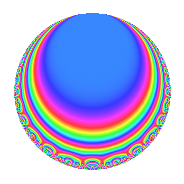Properties

 Label 6021.2.a.kLevel 6021 Weight 2 Character orbit 6021.a Self dual Yes Analytic conductor 48.078 Analytic rank 1 Dimension 4 CM No Inner twists 2

Related objects

Newspace parameters

 Level: $$N$$ = $$6021 = 3^{3} \cdot 223$$ Weight: $$k$$ = $$2$$ Character orbit: $$[\chi]$$ = 6021.a (trivial)

Newform invariants

 Self dual: Yes Analytic conductor: $$48.077927057$$ Analytic rank: $$1$$ Dimension: $$4$$ Coefficient field: $$\Q(\sqrt{2}, \sqrt{5})$$ Coefficient ring: $$\Z[a_1, \ldots, a_{5}]$$ Coefficient ring index: $$2$$ Fricke sign: $$1$$ Sato-Tate group: $\mathrm{SU}(2)$

$q$-expansion

Coefficients of the $$q$$-expansion are expressed in terms of a basis $$1,\beta_1,\beta_2,\beta_3$$ for the coefficient ring described below. We also show the integral $$q$$-expansion of the trace form.

 $$f(q)$$ $$=$$ $$q$$ $$+ \beta_{2} q^{2}$$ $$+ ( -\beta_{1} + \beta_{2} ) q^{5}$$ $$+ q^{7}$$ $$-2 \beta_{2} q^{8}$$ $$+O(q^{10})$$ $$q$$ $$+ \beta_{2} q^{2}$$ $$+ ( -\beta_{1} + \beta_{2} ) q^{5}$$ $$+ q^{7}$$ $$-2 \beta_{2} q^{8}$$ $$+ ( 1 - \beta_{3} ) q^{10}$$ $$+ ( -2 \beta_{1} + 2 \beta_{2} ) q^{11}$$ $$+ q^{13}$$ $$+ \beta_{2} q^{14}$$ $$-4 q^{16}$$ $$+ ( 2 \beta_{1} - 3 \beta_{2} ) q^{17}$$ $$+ 3 \beta_{3} q^{19}$$ $$+ ( 2 - 2 \beta_{3} ) q^{22}$$ $$+ ( 3 \beta_{1} - 4 \beta_{2} ) q^{23}$$ $$+ ( -2 - \beta_{3} ) q^{25}$$ $$+ \beta_{2} q^{26}$$ $$+ ( 3 \beta_{1} - 3 \beta_{2} ) q^{29}$$ $$+ ( -5 + \beta_{3} ) q^{31}$$ $$+ ( -4 + 2 \beta_{3} ) q^{34}$$ $$+ ( -\beta_{1} + \beta_{2} ) q^{35}$$ $$-9 q^{37}$$ $$+ ( 6 \beta_{1} - 3 \beta_{2} ) q^{38}$$ $$+ ( -2 + 2 \beta_{3} ) q^{40}$$ $$+ ( \beta_{1} + 2 \beta_{2} ) q^{41}$$ $$+ ( 4 - 2 \beta_{3} ) q^{43}$$ $$+ ( -5 + 3 \beta_{3} ) q^{46}$$ $$+ ( -2 \beta_{1} + 8 \beta_{2} ) q^{47}$$ $$-6 q^{49}$$ $$+ ( -2 \beta_{1} - \beta_{2} ) q^{50}$$ $$+ ( -\beta_{1} - 6 \beta_{2} ) q^{53}$$ $$+ ( 6 - 2 \beta_{3} ) q^{55}$$ $$-2 \beta_{2} q^{56}$$ $$+ ( -3 + 3 \beta_{3} ) q^{58}$$ $$+ ( 6 \beta_{1} - 6 \beta_{2} ) q^{59}$$ $$+ ( -4 - 3 \beta_{3} ) q^{61}$$ $$+ ( 2 \beta_{1} - 6 \beta_{2} ) q^{62}$$ $$+ 8 q^{64}$$ $$+ ( -\beta_{1} + \beta_{2} ) q^{65}$$ $$-9 q^{67}$$ $$+ ( 1 - \beta_{3} ) q^{70}$$ $$+ ( 6 \beta_{1} - 5 \beta_{2} ) q^{71}$$ $$+ ( 2 - 5 \beta_{3} ) q^{73}$$ $$-9 \beta_{2} q^{74}$$ $$+ ( -2 \beta_{1} + 2 \beta_{2} ) q^{77}$$ $$+ ( 8 + \beta_{3} ) q^{79}$$ $$+ ( 4 \beta_{1} - 4 \beta_{2} ) q^{80}$$ $$+ ( 5 + \beta_{3} ) q^{82}$$ $$+ ( -5 \beta_{1} + 5 \beta_{2} ) q^{83}$$ $$+ ( -7 + 3 \beta_{3} ) q^{85}$$ $$+ ( -4 \beta_{1} + 6 \beta_{2} ) q^{86}$$ $$+ ( -4 + 4 \beta_{3} ) q^{88}$$ $$+ ( 2 \beta_{1} - 2 \beta_{2} ) q^{89}$$ $$+ q^{91}$$ $$+ ( 14 - 2 \beta_{3} ) q^{94}$$ $$+ ( 3 \beta_{1} - 9 \beta_{2} ) q^{95}$$ $$+ ( -4 - 3 \beta_{3} ) q^{97}$$ $$-6 \beta_{2} q^{98}$$ $$+O(q^{100})$$ $$\operatorname{Tr}(f)(q)$$ $$=$$ $$4q$$ $$\mathstrut +\mathstrut 4q^{7}$$ $$\mathstrut +\mathstrut O(q^{10})$$ $$4q$$ $$\mathstrut +\mathstrut 4q^{7}$$ $$\mathstrut +\mathstrut 4q^{10}$$ $$\mathstrut +\mathstrut 4q^{13}$$ $$\mathstrut -\mathstrut 16q^{16}$$ $$\mathstrut +\mathstrut 8q^{22}$$ $$\mathstrut -\mathstrut 8q^{25}$$ $$\mathstrut -\mathstrut 20q^{31}$$ $$\mathstrut -\mathstrut 16q^{34}$$ $$\mathstrut -\mathstrut 36q^{37}$$ $$\mathstrut -\mathstrut 8q^{40}$$ $$\mathstrut +\mathstrut 16q^{43}$$ $$\mathstrut -\mathstrut 20q^{46}$$ $$\mathstrut -\mathstrut 24q^{49}$$ $$\mathstrut +\mathstrut 24q^{55}$$ $$\mathstrut -\mathstrut 12q^{58}$$ $$\mathstrut -\mathstrut 16q^{61}$$ $$\mathstrut +\mathstrut 32q^{64}$$ $$\mathstrut -\mathstrut 36q^{67}$$ $$\mathstrut +\mathstrut 4q^{70}$$ $$\mathstrut +\mathstrut 8q^{73}$$ $$\mathstrut +\mathstrut 32q^{79}$$ $$\mathstrut +\mathstrut 20q^{82}$$ $$\mathstrut -\mathstrut 28q^{85}$$ $$\mathstrut -\mathstrut 16q^{88}$$ $$\mathstrut +\mathstrut 4q^{91}$$ $$\mathstrut +\mathstrut 56q^{94}$$ $$\mathstrut -\mathstrut 16q^{97}$$ $$\mathstrut +\mathstrut O(q^{100})$$

Basis of coefficient ring in terms of a root $$\nu$$ of $$x^{4}\mathstrut -\mathstrut$$ $$6$$ $$x^{2}\mathstrut +\mathstrut$$ $$4$$:

 $$\beta_{0}$$ $$=$$ $$1$$ $$\beta_{1}$$ $$=$$ $$\nu$$ $$\beta_{2}$$ $$=$$ $$($$$$\nu^{3} - 4 \nu$$$$)/2$$ $$\beta_{3}$$ $$=$$ $$\nu^{2} - 3$$
 $$1$$ $$=$$ $$\beta_0$$ $$\nu$$ $$=$$ $$\beta_{1}$$ $$\nu^{2}$$ $$=$$ $$\beta_{3}\mathstrut +\mathstrut$$ $$3$$ $$\nu^{3}$$ $$=$$ $$2$$ $$\beta_{2}\mathstrut +\mathstrut$$ $$4$$ $$\beta_{1}$$

Embeddings

For each embedding $$\iota_m$$ of the coefficient field, the values $$\iota_m(a_n)$$ are shown below.

For more information on an embedded modular form you can click on its label.

Label $$\iota_m(\nu)$$ $$a_{2}$$ $$a_{3}$$ $$a_{4}$$ $$a_{5}$$ $$a_{6}$$ $$a_{7}$$ $$a_{8}$$ $$a_{9}$$ $$a_{10}$$
1.1
 0.874032 −2.28825 2.28825 −0.874032
−1.41421 0 0 −2.28825 0 1.00000 2.82843 0 3.23607
1.2 −1.41421 0 0 0.874032 0 1.00000 2.82843 0 −1.23607
1.3 1.41421 0 0 −0.874032 0 1.00000 −2.82843 0 −1.23607
1.4 1.41421 0 0 2.28825 0 1.00000 −2.82843 0 3.23607
 $$n$$: e.g. 2-40 or 990-1000 Significant digits: Format: Complex embeddings Normalized embeddings Satake parameters Satake angles

Inner twists

Char. orbit Parity Mult. Self Twist Proved
1.a Even 1 trivial yes
3.b Odd 1 yes

Atkin-Lehner signs

$$p$$ Sign
$$3$$ $$-1$$
$$223$$ $$-1$$

Hecke kernels

This newform can be constructed as the intersection of the kernels of the following linear operators acting on $$S_{2}^{\mathrm{new}}(\Gamma_0(6021))$$:

 $$T_{2}^{2}$$ $$\mathstrut -\mathstrut 2$$ $$T_{5}^{4}$$ $$\mathstrut -\mathstrut 6 T_{5}^{2}$$ $$\mathstrut +\mathstrut 4$$